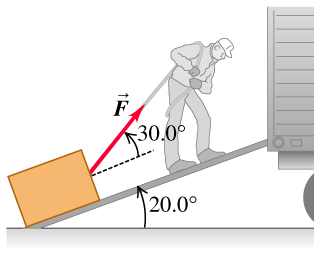# Problem: A man is dragging a trunk up the loading ramp of a moving truck. The ramp has a slope angle of 20.0°, and the man pulls upward with a force whose direction makes an angle of 30.0° with the ramp in the figure.(a) How large a force exttip{vec{F}}{F_vec} is necessary for the component exttip{F_{mit x}}{F_x} parallel to the ramp to be 90.0 N?(b) How large will the component exttip{F_{mit y}}{F_y} perpendicular to the ramp then be?

###### FREE Expert Solution
96% (370 ratings)
###### Problem Details

A man is dragging a trunk up the loading ramp of a moving truck. The ramp has a slope angle of 20.0°, and the man pulls upward with a force whose direction makes an angle of 30.0° with the ramp in the figure.
(a) How large a force is necessary for the component parallel to the ramp to be 90.0 N?
(b) How large will the component perpendicular to the ramp then be?Frequently Asked Questions

What scientific concept do you need to know in order to solve this problem?

Our tutors have indicated that to solve this problem you will need to apply the Vector Composition & Decomposition concept. You can view video lessons to learn Vector Composition & Decomposition. Or if you need more Vector Composition & Decomposition practice, you can also practice Vector Composition & Decomposition practice problems.

What professor is this problem relevant for?

Based on our data, we think this problem is relevant for Professor Jerousek's class at UCF.